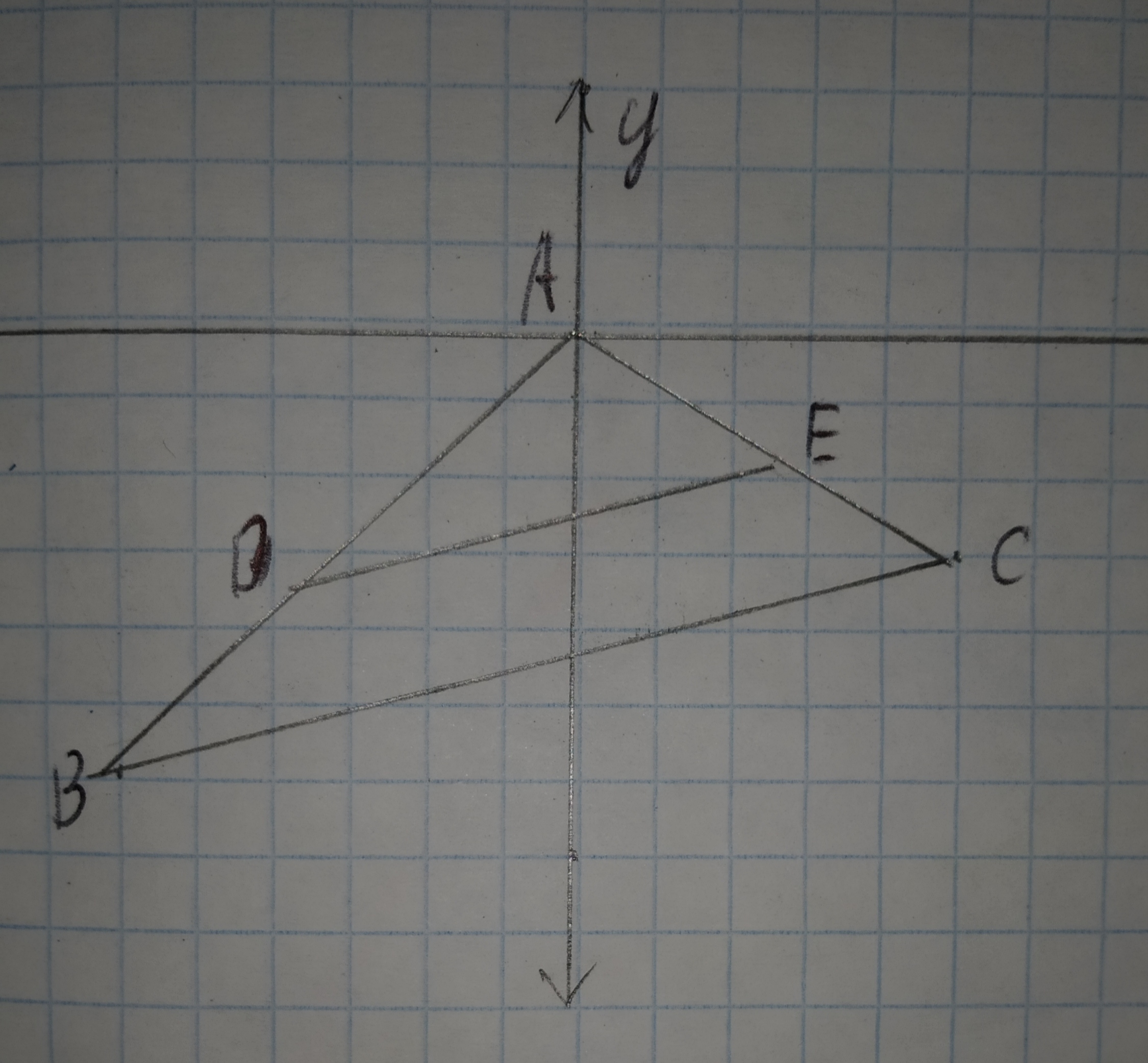# When is a rank one matrix diagonalizable? Justify your answer. What is one choice of the diagonalizing similarity? What happens when it is not diagonalizable? Justify your answer.Question
SimilarityWhen is a rank one matrix diagonalizable? Justify your answer. What is one choice of the diagonalizing similarity? What happens when it is not diagonalizable? Justify your answer.2021-03-10
Step 1
Let, the rank of (A−3I) is 1. A matrix is diagonalizable if for each eigen value $$\displaystyle\lambda$$, the rank r of the matrix
(A−3I)=n-the multiplicity of $$\displaystyle\lambda$$ , where n= size of the matrix
Step 2
A $$\displaystyle{n}\times{n}$$ matrix A is diagonalizable if it is similar to a diagonal matrix, that is, if there exists an invertible $$\displaystyle{n}\times{n}$$ matrix c and a diagonal matrix D such that $$\displaystyle{A}={C}{D}{C}^{{−{1}}}$$
Example:
$$\displaystyle{A}={\left[\begin{array}{ccc} {1}&{0}&{0}\\{0}&{5}&{0}\\{0}&{0}&{6}\end{array}\right]}={I}_{{3}}{\left[\begin{array}{ccc} {1}&{0}&{0}\\{0}&{5}&{0}\\{0}&{0}&{6}\end{array}\right]}{{I}_{{3}}^{{-{{1}}}}}$$
Ay diagonal matrix A is diagonalizable as it is similar to itself.
Step 3
If the matrix is not diagonalizable, the one has to find a matrix with same properties consisting of eigen values on the leading diagonal and either ones or zeros on the super diagonal.

### Relevant QuestionsCompare and contrast the Borda count method and the purality-with-elimination method. Identify at least one similarity and one difference. Use a specific example of voting preferences to illustrate your point.Finding a Lenght Two vertical poles, one 8 ft tall and the other 24 ft tall, have ropes stretched from the top of each to the base of the other (see the figure). How high above the ground is the point where the ropes cross? [Hint: Use similarity]Describe one similarity and one difference between the definitions of $$\displaystyle{\sin{{0}}}{\quad\text{and}\quad}{\cos{{0}}}$$, where 0 is an acute angle of a right triangle.Describe one similarity and one difference between the definitions of $$\displaystyle{\sin{{0}}},{\quad\text{and}\quad}{\cos{{0}}}$$, where 0 is an acute angle of a right triangle.Show the similarity $$\displaystyle\int\frac{{{x}}}{{{\left({x}^{{2}}+{a}^{{2}}\right)}^{{m}}}}{\left.{d}{x}\right.}=\frac{{{1}}}{{{2}{\left(-{m}+{1}\right)}{\left({x}^{{2}}+{a}^{{2}}\right)}^{{{m}-{1}}}}}+{C}$$ using the substitution $$\displaystyle{u}={x}^{{2}}+{a}^{{2}}$$. Also check that the integration is correct by deriving the answer.Draw a graph for the original figure and its dilated image. Check whether the dilation is a similarity transformation or not.
Given:
The given vertices are
J(-6,8),K(6,6),L(-2,4),D(-12,16),G(12,12),H(-4,8)Draw a graph for the original figure and its dilated image. Check whether the dilation is a similarity transformation or not.
Given:
The given vertices are
V(-3,4),W(-5,0),X(1,2),Y(-6,-2),Z(3,1)Draw a graph for the original figure and its dilated image. Check whether the dilation is a similarity transformation or not.
Given:
The given vertices are
original $$\displaystyle\rightarrow{A}{\left({2},{3}\right)},{B}{\left({0},{1}\right)},{C}{\left({3},{0}\right)}$$
image $$\displaystyle\rightarrow{D}{\left({4},{6}\right)},{F}{\left({0},{2}\right)},{G}{\left({6},{0}\right)}$$